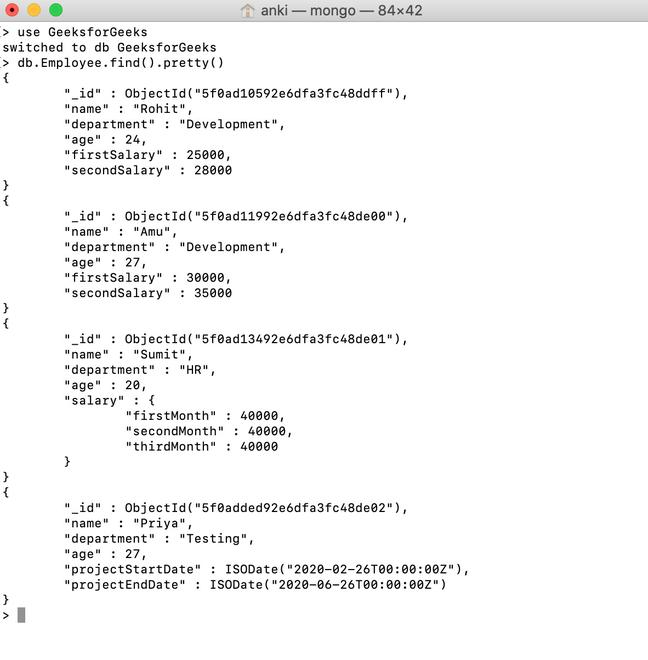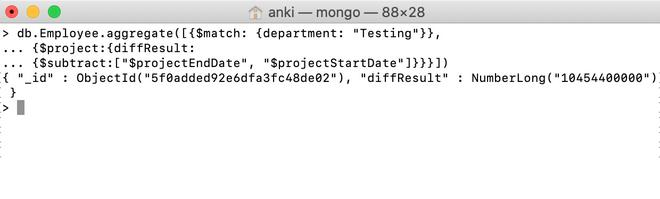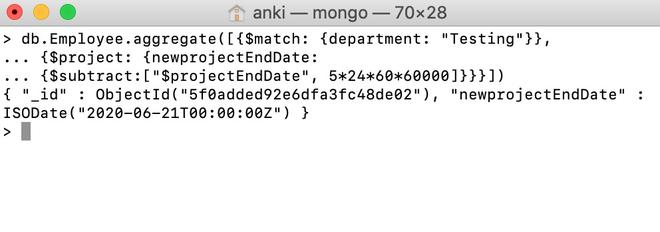GFG App
Open AppBrowser
Continue

MongoDB provides different types of arithmetic expression operators that are used in the aggregation pipeline stages and an \$subtract operator is one of them. This operator is used to subtract two numbers and return the difference in the numbers or to subtract two dates and return the difference in the milliseconds, or subtracts date and number in milliseconds and returns the date.

Syntax:

`{ \$subtract: [ <expression1>, <expression2> ] }`

Here, the given arguments must be a valid expression like numbers or a date, and the second argument is subtracted from the first argument.  If you are subtracting a number from a date, then the first argument of this operator is a date.

Examples:

In the following examples, we are working with:

Database: GeeksforGeeks

Collection: Employee

Document: four documents that contain the details of the employees in the form of field-value pairs.Using \$subtract operator subtract two numbers:

In this example, we are going to subtract the value of firstSalary field from the value of secondSalary field using \$subtract operator.

```db.Employee.aggregate([{\$match: {department: "Development"}},
... {\$project: {result:
... {\$subtract:["\$secondSalary", "\$firstSalary"]}}}])```Using \$subtract operator subtract two dates:

In this example, we are going to subtract two dates, i.e, the value of projectStartDate field from the value of projectEndDate field using a \$subtract operator.

```db.Employee.aggregate([{\$match: {department: "Testing"}},
... {\$project:{diffResult:
... {\$subtract:["\$projectEndDate", "\$projectStartDate"]}}}])```Using \$subtract operator subtracts milliseconds from a date:

In this example, we are going to subtract 5*24*60*60000 milliseconds (i.e., 5 days) from the value of projectEndDate field using a \$subtract operator.

```db.Employee.aggregate([{\$match: {department: "Testing"}},
... {\$project: {newprojectEndDate:
... {\$subtract:["\$projectEndDate", 5*24*60*60000]}}}])```My Personal Notes arrow_drop_up